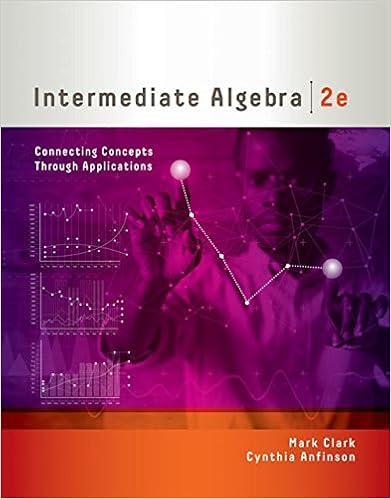# 5 evaluate the expressions below for x 1 and y 2 a 3

• Test Prep
• 6
• 67% (3) 2 out of 3 people found this document helpful

This preview shows page 3 - 6 out of 6 pages.

##### We have textbook solutions for you!
The document you are viewing contains questions related to this textbook.The document you are viewing contains questions related to this textbook.
Chapter 7 / Exercise 1
Intermediate Algebra: Connecting Concepts through Applications
ClarkExpert Verified
5) Evaluate the expressions below for x = -1 and y = -2 a) 3 2 2 x y b) 2 5 10 x y 6) Solve the equation below and show the check of your answer. : 2
##### We have textbook solutions for you!
The document you are viewing contains questions related to this textbook.The document you are viewing contains questions related to this textbook.
Chapter 7 / Exercise 1
Intermediate Algebra: Connecting Concepts through Applications
ClarkExpert Verified
Math 009 Quiz 1 Page 4 7) Solve the equation below and show the check of your answer. 52 4 w   8) Solve the equation below and show the check of your answer. 10 6 n
Math 009 Quiz 1 Page 5 9) The top of a mountain has an altitude of 14,250 feet above sea level and the bottom of a valley nearby is at 113 feet below sea level. Answer each of the following questions, including units with all answers. a) Find the difference between these two elevations. b) Round your answer from part (a) to the nearest 10 feet. c) Round your answer from part (a) to the nearest 100 feet. d) Round your answer from part (a) to the nearest 1000 feet. e) Round your answer from part (a) to the nearest 10,000 feet.
Math 009 Quiz 1 Page 6 10) Jackson’s scores on the first four algebra quizzes are 80, 82, 95, and 61. What score must he earn on the fifth quiz to earn an average quiz score of 80? Please set up and solve this problem following the steps below: a) Define the unknown quantity in terms of one variable. b) Translate the question into an equation. c) Solve the equation, showing all work. d) Write a complete answer to the question asked. End of quiz: please do not forget to write and sign (or type) the required statement explained in the box on page 1 of the quiz.
•••